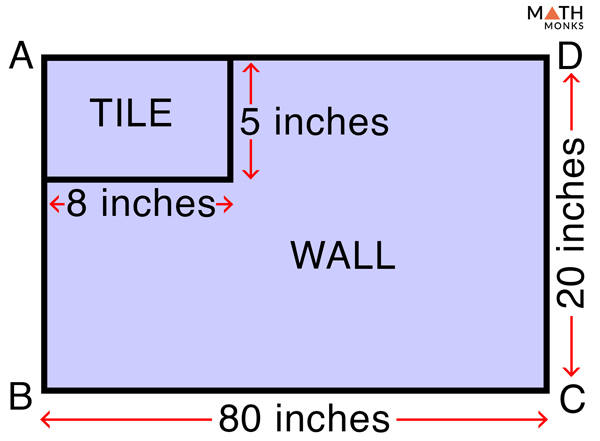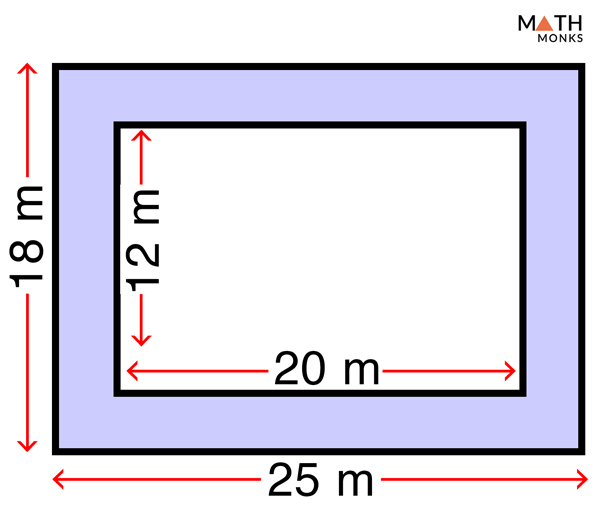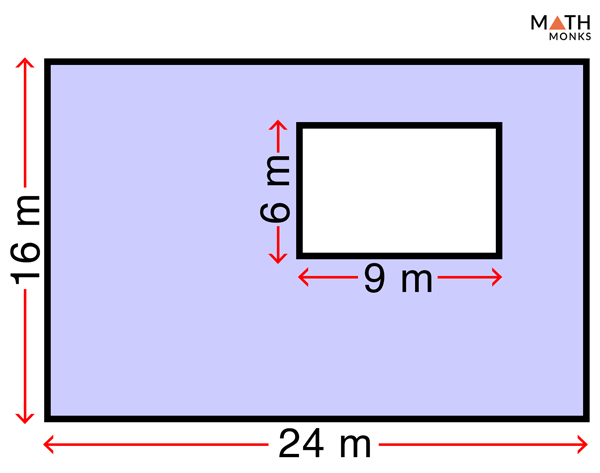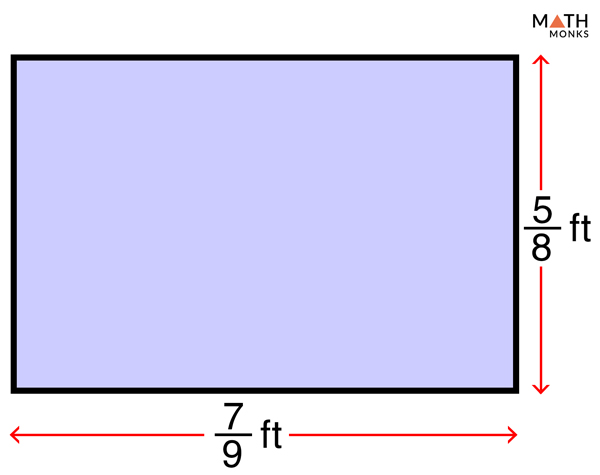# Area of Rectangle

A rectangle is a 2-dimensional shape having four sides. In this article, we will discuss the area of a rectangle and how we calculate it.

## What is the Area of Rectangle

The area of a rectangle is defined as the total region covered by it. It is the product of its 2 adjacent sides, i.e., length and width. We measure it in square units.

## Formulas

The formula to find the area of a rectangle is given below:

### How to Find the Area of a Rectangle

There are 3 standard steps to calculate the area of a rectangle given its length and width.

Step 1: Note the value and units of length and width from the given question or example

Step 2: Multiply length and width

Step 3: Write down the answer in square units

Now we will derive the standard formula for the area of a rectangle.

### Derivation: Why Area of the Rectangle is Width × Length

We take a ▭ ABCD and draw a diagonal BD.

It clearly divides the rectangle into 2 equivalent right-angled triangles ∆BAD and ∆BCD.

Therefore, the area of the rectangle will be the sum of the area of these two triangles.

As we know that ∆BAD and ∆BCD are congruent right-angled triangles

Area of ▭ ABCD = Area of ∆BAD + Area of ∆BCD

= 2 × area of ∆BCD

= 2 × (1/2 × base × height of ∆BCD)

= (CD × BC)

When CD = w, BC = l

Area of ▭ ABCD = w × l = width × length

Thus we get the standard formula of the area of a rectangle given its length and width.

Now let us solve few examples using the above formula to calculate the area of a rectangle.

Find the area of a rectangular swimming pool 45 feet long and 25 feet wide.

Solution:

As we know,
Area (A) = w × l, here, w = 25 ft, and l = 45 ft
= (25 × 45) sq. ft
= 1125 sq. ft.

Dividing a Rectangle into Unit Squares

Why is the area expressed in square units?

Let us take a rectangle of length 3 inches and width 2inches.

Now we draw unit squares inside the rectangle.

Each unit square is of length 1 inch.

Now, count the number of squares in the rectangular block.

There are 6 squares inside it.

We always measure the area in square units.

Since the unit of this rectangle is ‘inches’, the area is measured and expressed in square inches.

Thus, the area of the above rectangle is 6 square inches.Find the number of tiles needed to cover a rectangular wall 20 inches wide and 80 inches long. Each tile is 8 inches long and 5 inches wide.

Solution:

As we know,
Area of wall = w × l, here, w= 20 inches , and l = 80 inches
= (20 × 80) sq. inches
= 1600 sq. inches
Area of tile = w × l, here, w= 5 inches , and l = 8 inches
= (5 × 8) sq. inches
= 40 sq. inches.
Therefore, the number of tiles needed to cover the wall = area of the rectangular wall ÷ area of a rectangular tile
= 1600 ÷ 40 = 40
So we will need 40 tiles to cover the wall.

Find the number of tiles needed to cover a floor 3630 sq. inches with square tiles each having side of 11 inches.

Solution:

As we know,
Area of square tile  = a2, here, a = 11 inches
= 11 × 11
= 121 sq. inches.
Number of tiles required = area of floor ÷ area of tile, here area of floor = 3630 sq. inches
3630 ÷ 121 = 30
So 30 tiles are needed to cover the floor.

Besides the standard way of finding the area of a rectangle, let us calculate the area, given the diagonal and any one of its sides, i.e., the length or width.

### Area of a Rectangle When Diagonal and Any One Side is Given

The formula to calculate the area of a rectangle is derived below.

#### Derivation

As we know from the Pythagorean theorem,

(Diagonal)2 = (Length)2 + (Width)2,

Here, in ▭ ABCD, diagonal = d, length = l, width = w

Therefore, l2 = d2 – w2 ,

or, l = √(d2 – w2) ……….(1)

Alternatively, w2 =  d2 – l2

or, w = √(d2 – l2) ……….(2)

We know that, area  of rectangle (A) = w × l

There can be two possibilities:

1. Diagonal and width are known

Substituting the value of l from (1) in the standard formula, we get,

A = w × √(d2 – w2)

2. Diagonal and length are known

Substituting the value of w from (2) in the standard formula, we get,

A = l × (d2 – l2)

Now we will solve few examples involving the above formulas of diagonal and side.

Finding the area of a rectangle when the DIAGONAL and ONE SIDE are known

Find the area of a rectangle whose diagonal is 15 cm and length is 12 cm.

Solution:

As we know,
Area of rectangle A = l × √(d2 – l2), here d = 15 cm, l = 12 cm
= 12 × √(225 – 144) sq. cm
= (12 × 9) sq. cm = 108 sq. cm

Find the area of a rectangle whose diagonal is 19 cm and width is 9 cm.

Solution:

As we know,
Area of rectangle A = w × √(d2 – w2), here d = 19 cm, w = 9 cm
= 9 × √(192 – 92) sq. cm
≈ 150.6 sq. cmFind the area of the shaded region.

Solution:

As we know,
Area (A) = w × l, here, w = 18 m, and l = 25 m for the bigger rectangle
= (18 × 25) sq. m
= 450 sq. m
And,
Area (A) = w × l, here, w = 12 m, and l = 20 m for the smaller rectangle
= (12 × 20) sq. m
= 240 sq. m
Now,
The area of the shaded region = Area of the bigger rectangle – Area of the smaller rectangle
= (450 – 240) sq. m
= 210 sq. mFind the area of the shaded region.

Solution:

As we know,
Area (A) = w × l, here, w = 16 m, and l = 24 m for the bigger rectangle
= (16 × 24) sq. m
= 384 sq. m
Similarly,
Area (A) = w × l, here, w = 6 m, and l = 9 m for the smaller rectangle
= (6 × 9) sq. m
= 54 sq. m
Now,
The area of the shaded region = Area of the bigger rectangle – Area of the smaller rectangle
= (384 – 54) sq. m
= 330 sq. mFind the area of a rectangle with fractional side lengths of 7/9 and 5/8 of a feet.

Solution:

As we know,
Area (A) = w × l, here, w = 5/8 ft, and l = 7/9 ft
= 5/8 × 7/9 sq. ft
= 35/72 sq. ft
Thus, we get the area of a rectangle in fraction form when the side lengths are given in fraction form.Find the area of a rectangle with sides 1 and 1of a yard.

Solution:

As we know,
Area (A) = w × l, here, w= 1 yd and l = 1 yd
= 1× 1
=  ×   sq. yd
=  sq. yd
≈ 2.058 sq. yd

Find the maximum area of a rectangle with a fixed perimeter? It has a length of 120 cm and a width of 40 cm.

Solution:

As we know,
Area (A) of rectangle = w × l, here w = 40 cm, l = 120 cm
= 40 × 120
= 4800 sq. cm.
Perimeter (P) of the rectangle = 2 (w + l),
= 2 × (120 + 40)
= 320 cm.
Now, let’s take a square with a perimeter (P) same as the rectangle, i.e., 320 cm.
As we know,
a = P/4, here P = 320 cm, a = each side of a square
= 320/4
= 80 cm
And, area (A) of square = a2
= 80 × 80
= 6400 sq. cm
Thus, if we want to get the maximum area of a rectangle with a fixed perimeter, we must convert it into a square.

## FAQs

Q1. How to find the maximum area of a rectangle with a fixed perimeter

Ans. To attain the maximum area of a rectangle, one must have all its sides equal. In other words, to optimize the area of a rectangle with a fixed perimeter, one needs to convert it into a square.